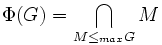# Equivalence of definitions of Frattini subgroup

This article gives a proof/explanation of the equivalence of multiple definitions for the term Frattini subgroup
View a complete list of pages giving proofs of equivalence of definitions

## The definitions that we have to prove as equivalent

### Definition in terms of maximal subgroups

For a group$G$, the Frattini subgroup$\Phi(G)$ is defined as the intersection of all maximal subgroups of$G$:$\Phi(G) = \bigcap_{M \le_{max} G} M$

When there are no maximal subgroups, we define$\Phi(G)$ as the whole group$G$

### Definition as the set of nongenerators

For a group$G$, the Frattini subgroup$\Phi(G)$ is defined as the set of all nongenerators. An element$x \in G$ is termed a nongenerator if, whenever$S \subset G$ is such that$S \cup \{ x \}$ generates$G$,$S$ itself generates$G$.

## Proof

### The set of nongenerators is contained in every maximal subgroup

Given: A group$G$, a nongenerator$x$ for$G$, a maximal subgroup$M$ of$G$

To prove:$x \in M$

Proof: Suppose (contradiction point)$x \notin M$. Then,$\langle M,x \rangle = G$. Thus, the set$M \cup \{ x \}$ is a generating set for$G$. By the definition of nongenerator, we see that$M$, as a set, is a generating set for$G$. But this is a contradiction since the subgroup generated by$M$ is$M$ itself.

Thus,$x \in M$ is forced.

### Any element in the intersection of all maximal subgroups is a nongenerator

Given: A group$G$, an element$x$ in the intersection of maximal subgroups of$G$, a subset$S$ such that$S \cup \{ x \}$ generates$G$

To prove:$S$ generates$G$

Proof: Let$H$ be the subgroup generated by$S$. Suppose (contradiction point)$H$ is proper. Then,$x \notin H$, since$\langle H,x \rangle = G$. Thus,$H$ is a 1-completed subgroup: it, along with one outside element, generates the whole group. By the above fact, there exists a maximal subgroup$M$ containing$H$. But by assumption,$x$ is in every maximal subgroup, so$x \in M$, so$\langle H,x \rangle \le M$, so$G \le M$, a contradiction.

Thus, the assumption that$H$ is proper is false, so$H = G$, so$S$ generates$G$.